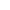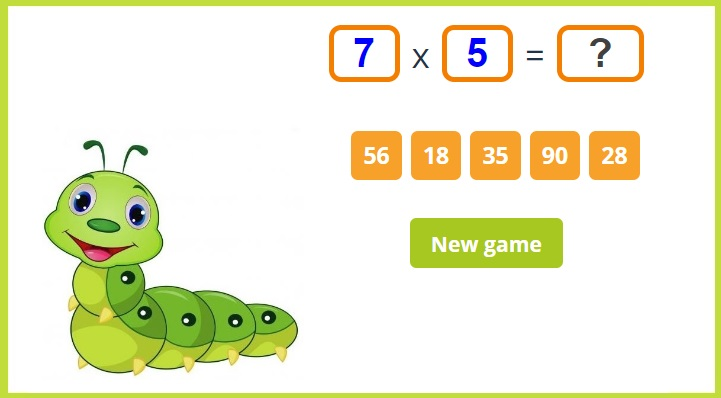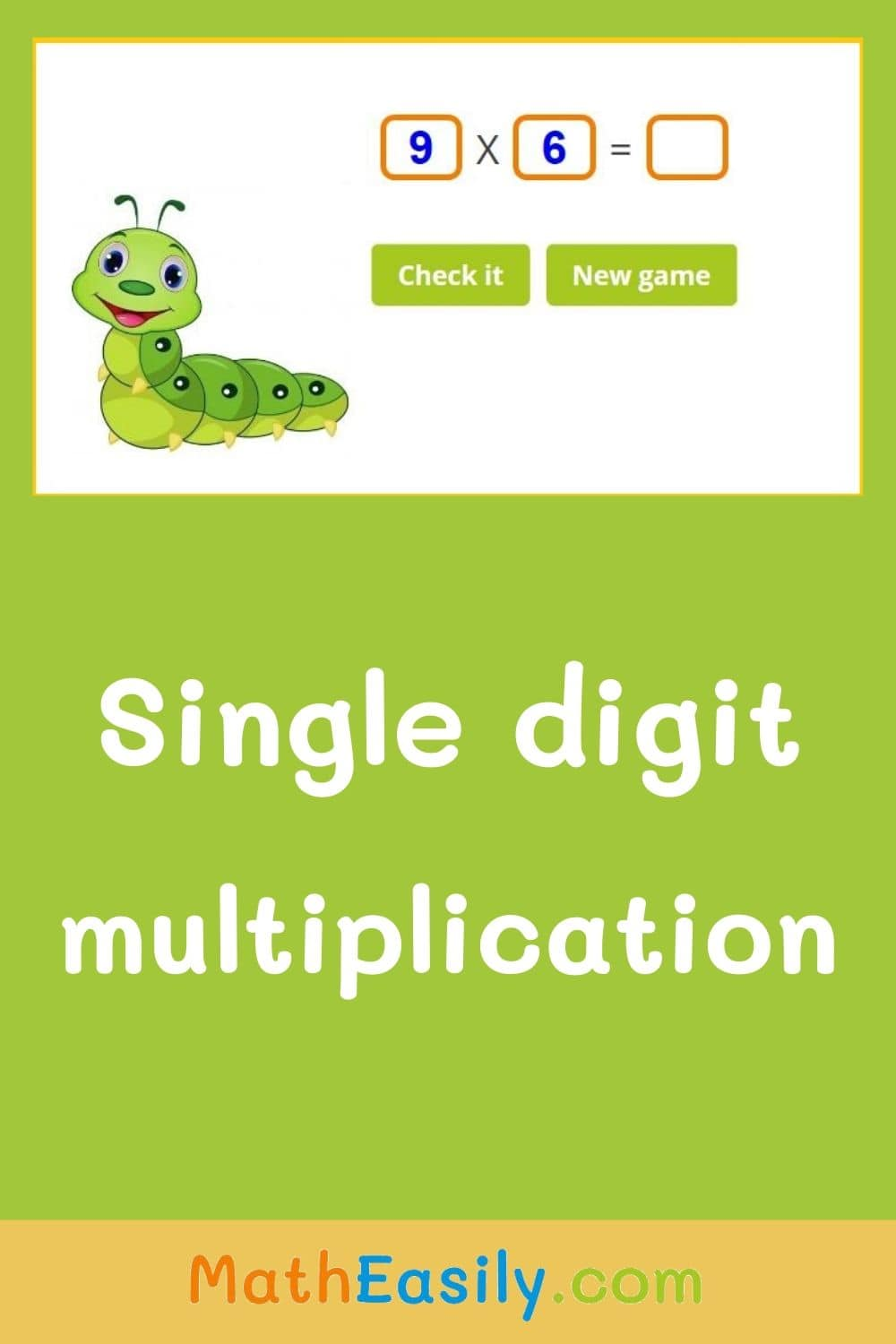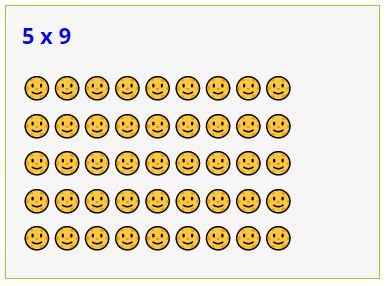﻿ Single Digit Multiplication Games + Worksheets

Page No. 1080

# Single digit multiplication games

Single digit multiplication games online. Free single digit multiplication worksheets printable free. Multiplication by single digit. One digit by one digit multiplication worksheet. One digit multiplication games printable. Single digit multiplication table. Multiplication worksheet single digit. single multiplication worksheets PDF. single digit multiplication practice online. single digit multiplication sums. multiplication one digit worksheets PDF. One digit by one digit multiplication with pictures.

x =
New gameSingle digit multiplication game: select the correct answer.

## Single digit multiplication games onlineSelect the correct answer of single digit multiplication. Press the button "New game" and play another one digit multiplication game.

### Printable single digit multiplication games

These online single digit multiplication games complement well with following printable single digit multiplication worksheets. A new original one digit multiplication worksheet is generated every time.

Click the picture bellow and generate the new printable single digit multiplication worksheet in PDF:

### One digit multiplication games

50 multiplication puzzles

Fun printable single digit multiplication games. 50 different pictures, many one digit multiplication tasks.

US\$ 3.00

## Free printable single digit multiplication worksheets in PDF

Here are some other free printable single digit multiplication worksheets in PDF, which you can download. You can find even more free multiplication worksheets here.

## Single digit multiplication practice online

This single digit multiplication game teaches basic rules of multiplication. You can play this game online. The multiplication table in PDF will help you to master it.

Do you want to practice single digit by single digit multiplication in another game? Then try this funny multiplication game with a dice, this multiplication pyramid puzzle, multiplication crosswords or these multiplication flash cards.Do you want to learn multiplication and comparing numbers at the same time? Then try this game: multiplication comparisons within 100.

Learn about the concept of single multiplication with the help of little fishes in this video.## Multiplication by a single digit

Successful mastery of single digit multiplication is a prerequisite for children to begin with:

You can practice 1 digit by 1 digit multiplication by playing our interactive single digit multiplication games or you can download our free printable single digit multiplication worksheets in PDF.

## One digit by one digit multiplication with pictures

Multiplication is a mathematical operation that represents repeated addition. It is often helpful to visualize multiplication using pictures, especially for younger kids.

Our single digit multiplication games use arrays to illustrate multiplication facts. A multiplication array is a rectangular grid of objects (or pictures), where each row and column represents a group of objects.

### Multiplication using pictures

For example, to represent the multiplication 5 x 9, we can draw an array with 5 rows and 9 columns, and fill each cell with a picture.

This array shows that we have 5 groups of 9 smiley faces each, which we can count to find the answer: 5 x 9 = 45.Pictures can help make multiplication more concrete and intuitive for learners of all ages.

## Tips for learning single multiplication

Here are some tips to learn single digit multiplication:

• Skip counting: 💡 Skip counting is a way to count by a certain number. For example, to count by twos, you would say 2, 4, 6, 8, and so on. Skip counting help children learn multiplication tables more quickly. You can use this online multiplication chart for learning skip counting.
• Commutative property: 💡 The commutative property of multiplication states that the order of the factors does not change the product. For example, 2 x 3 is the same as 3 x 2. This property can help children memorize multiplication tables more easily.
• Use visual aids: 💡 Visual aids such as multiplication tables, flashcards, and multiplication puzzles can help children learn multiplication more easily.

And a lot of practice. The key to mastering any skill is practice. Encourage children to practice single digit multiplication regularly to build fluency and confidence. These online single digit multiplication games are here for you!